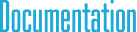#Point3d Class Reference

## Detailed Description

3D point (vector) class with double type elementsInheritance diagram for Point3d:

## Public Member Functions

Binary operators
Point3 operator+ (const Point3 &p) const

Point3 operator+ (const double &v) const

Point3 operator- (const Point3 &p) const

Point3 operator- (const double &v) const

Point3 operator* (const Point3 &p) const

Point3 operator* (const double &v) const

Point3 operator/ (const Point3 &p) const

Point3 operator/ (const double &v) const

Unary operators
Point3 operator- () const

Set & Get value methods
void Zero ()

void Get (double *p) const

void Set (const double *p)

void Set (const double &v)

void Set (const double &_x, const double &_y, const double &_z)

General methods
double LengthSquared () const

double Length () const

void Normalize ()

Point3 GetNormalized () const

double Sum () const

bool IsZero () const

double Min () const

double Max () const

int MinID () const

int MaxID () const

Limit methods
void Clamp (const double &minValue, const double &maxValue)

void ClampMin (const double &v)

void ClampMax (const double &v)

void Abs ()

Assignment operators
const Point3operator+= (const Point3 &p)

const Point3operator+= (const double &v)

const Point3operator-= (const Point3 &p)

const Point3operator-= (const double &v)

const Point3operator*= (const Point3 &p)

const Point3operator*= (const double &v)

const Point3operator/= (const Point3 &p)

const Point3operator/= (const double &v)

Test operators
bool operator== (const Point3 &p) const

bool operator!= (const Point3 &p) const

Access operators
double & operator[] (int i)

const double & operator[] (int i) const

double & Element (int i)

const double & Element (int i) const

double * Data ()

const double * Data () const

Cross product and dot product
Point3 Cross (const Point3 &p) const

Point3 operator^ (const Point3 &p) const

double Dot (const Point3 &p) const

double operator% (const Point3 &p) const

Conversion Methods
Point2< double > XY () const

## Public Attributes

Components of the point/vector
double x

double y

double z

## § Zero()

 void Zero ( )
inherited

Sets the coordinates as zero.

## § Get()

 void Get ( double * p ) const
inherited

Puts the coordinate values into the array.

## § Set() [1/3]

 void Set ( const double * p )
inherited

Sets the coordinates using the values in the given array.

## § Set() [2/3]

 void Set ( const double & v )
inherited

Sets all coordinates using the given value.

## § Set() [3/3]

 void Set ( const double & _x, const double & _y, const double & _z )
inherited

Sets the coordinates using the given values.

## § LengthSquared()

 double LengthSquared ( ) const
inherited

Returns the square of the length. Effectively, this is the dot product of the vector with itself.

## § Length()

 double Length ( ) const
inherited

Returns the length of the vector.

## § Normalize()

 void Normalize ( )
inherited

Normalizes the vector, such that its length becomes 1.

## § GetNormalized()

 Point3 GetNormalized ( ) const
inherited

Returns a normalized copy of the vector.

## § Sum()

 double Sum ( ) const
inherited

Returns the sum of its components.

## § IsZero()

 bool IsZero ( ) const
inherited

Returns true if all components are exactly zero.

## § Min()

 double Min ( ) const
inherited

Returns the square of the length. Effectively, this is the dot product of the vector with itself.

## § Max()

 double Max ( ) const
inherited

Returns the square of the length. Effectively, this is the dot product of the vector with itself.

## § MinID()

 int MinID ( ) const
inherited

Returns the square of the length. Effectively, this is the dot product of the vector with itself.

## § MaxID()

 int MaxID ( ) const
inherited

Returns the square of the length. Effectively, this is the dot product of the vector with itself.

## § Clamp()

 void Clamp ( const double & minValue, const double & maxValue )
inherited

Converts all negative components to positive values.

## § ClampMin()

 void ClampMin ( const double & v )
inherited

Converts all negative components to positive values.

## § ClampMax()

 void ClampMax ( const double & v )
inherited

Converts all negative components to positive values.

## § Abs()

 void Abs ( )
inherited

Converts all negative components to positive values.

## § Cross()

 Point3 Cross ( const Point3< double > & p ) const
inherited

Cross product.

## § operator^()

 Point3 operator^ ( const Point3< double > & p ) const
inherited

Cross product.

## § Dot()

 double Dot ( const Point3< double > & p ) const
inherited

Dot product.

## § operator%()

 double operator% ( const Point3< double > & p ) const
inherited

Dot product.